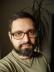## 3 Sum

Given an array `@L` of integers. Write a script to find all unique triplets such that a + b + c is same as the given target T. Also make sure a <= b <= c.

Example:

``@L = (-25, -10, -7, -3, 2, 4, 8, 10);``

One such triplet for target 0 i.e. -10 + 2 + 8 = 0.

I hadn’t checked the wiki page before writing my solution; and I hadn’t changed the solution after I read it. Therefore, it presents the naive and inefficient solution that iterates over all the possible triplets (but not starting from 0 in the inner loops to avoid checking the same triplet several times).

``````#!/usr/bin/perl
use warnings;
use strict;
use feature qw{ say };

my @L = (-25, -10, -7, -3, 2, 4, 8, 10);
my \$target = 0;

for my \$i (0 .. \$#L - 2) {
for my \$j (\$i + 1 .. \$#L - 1) {
for my \$k ( \$j + 1 .. \$#L) {
say join ' + ', sort { \$a <=> \$b } @L[\$i, \$j, \$k]
if \$target == \$L[\$i] + \$L[\$j] + \$L[\$k];
}
}
}``````

## Colourful Number

Write a script to display all Colourful Numbers with 3 digits.

A number can be declared Colourful Number where all the products of consecutive subsets of digit are different.

For example, 263 is a Colourful Numberb since 2, 6, 3, 2×6, 6×3, 2×6×3 are unique.

To get all the consecutive subsets, we need to inspect all the substrings (not subsequences, as they don’t have to be consecutive) starting at each position.

Let’s implement it as two nested loops, the outer one looping over the possible lengths of the substring, the inner one looping over the starting positions.

The pattern describing the subset is then an array of zeros before the position and ones starting at position, repeated length times. We can then use grep to map the pattern to the original digits. We can store the product in a hash and at the end, check the number of different products, i.e. the number of keys in the hash. If all the products are unique, the number will equal the number of all the possible substrings.

If the length of the input is n, there is 1 substring of length n, it starts at position 0 and spans the whole input. For length n - 1, there are two substrings, starting at positions 0 and 1; and so on up to length 1 which can start at any position 0 .. n - 1. Therefore, there are 1 + 2 + … + n possible substrings, which can be expressed as (n + 1)n / 2 or (n2 + n) / 2.

``````#!/usr/bin/perl
use warnings;
use strict;
use feature qw{ say };

use List::Util qw{ product };

sub is_colourful_number {
my (\$n) = @_;
my \$max_length = length \$n;
my %uniq;
my \$count = 0;
for my \$length (1 .. \$max_length) {
for my \$pos (0 .. \$max_length - \$length) {
my @pattern = ((0) x \$pos, (1) x \$length);
undef \$uniq{
product((split //, \$n)[ grep \$pattern[\$_],
0 .. \$#pattern ])
};
}
}
return (\$max_length ** 2 + \$max_length) / 2 == keys %uniq;
}

say for grep is_colourful_number(\$_), 100 .. 999;``````I blog about Perl.